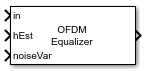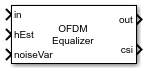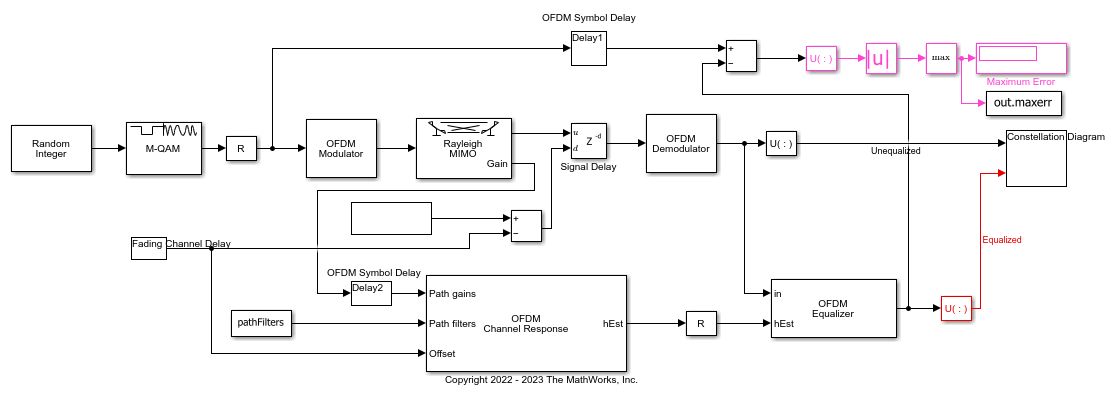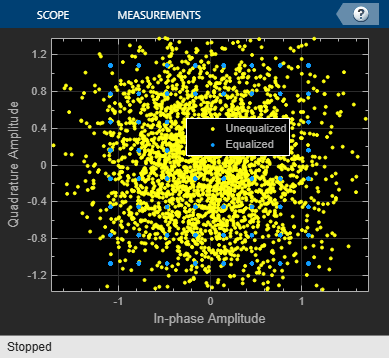# OFDM Equalizer

Equalize OFDM modulated signals

Since R2022b

•Libraries:
Communications Toolbox / Equalizers

## Description

The OFDM Equalizer block performs frequency-domain equalization to recover OFDM modulated symbols transmitted through a channel.

This icon shows the block with all ports enabled.## Examples

expand all

Apply equalization to an OFDM-modulated QAM signal that has been filtered through a Rayleigh MIMO channel.

The `cm_ofdm_equalization` model initializes the simulation variable in the `InitFun` callback function. For more information, see Model Callbacks (Simulink).The model generates random integer data, applies 64-QAM, and then applies OFDM to the QAM-modulated signal. The OFDM-modulated signal gets filtered through a MIMO Rayleigh fading channel. The model adds a signal delay, and then OFDM-demodulates the signal. In a parallel path, an OFDM Channel Response block computes the perfect OFDM channel response and an OFDM Equalizer block equalizes the received signal. A constellation diagram displays the unequalized and equalized signals. Compare the transmitted QAM signal and the equalized signal on the receive side to compute the error.

`The maximum computed error is 0.000558.`## Ports

### Input

expand all

Input signal, specified as a 3-D or 2-D array of received OFDM symbols.

• If Data format is set to `3-D`, the input signal must be specified as an NSC-by-NSymbols-by-NR array. NSC represents the number of OFDM subcarriers, NSymbols represents the number of OFDM symbols, and NR represents the number of receive antennas.

• If Data format is set to `2-D`, the input signal must be specified as an NRE-by-NR array. NRE represents the number of resource elements in an irregular subset of the OFDM subcarrier symbol grid.

Data Types: `double` | `single`
Complex Number Support: Yes

Channel estimate, specified as a 3-D array.

• If Data format is set to `3-D`, the block expects hEst to be an NSC-by-NT-by-NR or an (NSC×NSymbols)-by-NT-by-NR array.

• If hEst is an NSC-by-NT-by-NR array, all OFDM symbols in the input signal `in` are equalized by the same channel estimate. NSC represents the number of OFDM subcarriers, NT represents the number of transmit antennas, and NR represents the number of receive antennas.

• If hEst is an (NSC×NSymbols)-by-NT-by-NR array, each OFDM symbol in the input signal `in` is equalized by the corresponding entry in hEst. NSymbols represents the number of OFDM symbols.

• If Data format is set to `2-D`, the block expects hEst to be an NRE-by-NT-by-NR array. Each OFDM symbol in the input signal `in` is equalized by the corresponding entry in hEst. NRE represents the number of resource elements in an irregular subset of the OFDM subcarrier symbol grid.

Data Types: `double` | `single`
Complex Number Support: Yes

Noise variance estimate for minimum mean squared error (MMSE) equalization, specified as a nonnegative scalar.

#### Dependencies

The noise variance input port is used only when you set Noise variance source to ```Input port``` and Algorithm to `Minimum mean squared error`.

Data Types: `double` | `single`

### Output

expand all

Equalized symbols, returned as a 3-D or 2-D array.

• If Data format is set to `3-D`, the block returns an NSC-by-NSymbols-by-NT array. NSC represents the number of OFDM subcarriers, NSymbols represents the number of OFDM symbols, and NT represents the number of transmit antennas.

• If Data format is set to `2-D`, the block returns an NRE-by-NT array. NRE represents the number of resource elements in an irregular subset of the OFDM subcarrier symbol grid.

Soft channel state information, returned as a matrix with `size(csi,1) = size(hEst,1)` and ```size(csi,2) = NT = size(hEst,2)```. NT represents the number of transmit antennas.

#### Dependencies

To enable this output port, select the Output soft channel state information parameter.

## Parameters

expand all

To edit block parameters interactively, use the Property Inspector. From the Simulink® Toolstrip, on the Simulation tab, in the Prepare gallery, select .

Format of the signals, specified as `3-D` or `2-D`.

When this parameter is set to `3-D`, OFDM subcarriers and OFDM symbols use two separate dimensions in the representation of in and out.

• The in input must be an NSC-by-NSymbols-by-NR array.

• The out output is returned as an NSC-by-NSymbols-by-NT array.

When this parameter is set to `2-D`, OFDM subcarriers and OFDM symbols use one combined dimension in the representation of in and out.

• The in input must be an NRE-by-NR array.

• The out output is returned as an NRE-by-NT array.

NSC represents the number of OFDM subcarriers. NSymbols represents the number of symbols. NRE represents the number of resource elements in an irregular subset of the OFDM subcarrier symbol grid. NT represents the number of transmit antennas. NR represents the number of receive antennas.

Equalization algorithm, specified as ```Minimum mean squared error``` or `Zero-forcing`.

• When this parameter is set to ```Minimum mean squared error```, the block equalizes using the MMSE algorithm.

• When this parameter is set to `Zero-forcing`, the block equalizes using the zero-forcing algorithm. When using the zero-forcing algorithm, the noiseVar port value is ignored.

Source of noise variance, specified as one of these values:

• `Input port` — Specify this value to use the noiseVar input port to specify the noise variance estimate for MMSE equalization.

• `Property` — Specify this value to use the Noise variance parameter to specify the noise variance estimate for MMSE equalization.

Noise variance estimate for MMSE equalization, specified as a nonnegative scalar.

#### Dependencies

The noise variance setting is used only when you set Noise variance source to `Property` and Algorithm to ```Minimum mean squared error```.

Select this parameter to enable output port csi containing the soft channel state information.

Type of simulation to run, specified as ```Interpreted execution``` or `Code generation`.

• `Interpreted execution` — Simulate the model by using the MATLAB® interpreter. This option requires less startup time, but the speed of subsequent simulations is slower than with the ```Code generation``` option. In this mode, you can debug the source code of the block.

• `Code generation` — Simulate the model by using generated C code. The first time you run a simulation, Simulink generates C code for the block. The model reuses the C code for subsequent simulations unless the model changes. This option requires additional startup time, but the speed of the subsequent simulations is faster than with the `Interpreted execution` option.

## Block Characteristics

 Data Types `double` | `single` Multidimensional Signals `yes` Variable-Size Signals `yes`

## Version History

Introduced in R2022b